# Calculating Watts / Kw and KWh

Hi,
I have two sensors connected, voltage measured from Sonoff POW and a CT Clamp Current Sensor on nodemcu Esphome.

What I want to do is calculate both Watts / kw and KWh in HA and show / display calculated values on my glance card…

I think this can be achieved with a Template but unsure how to implement as my knowledge is very basic for now, something like this?

``````# Example configuration.yaml entry
sensor:
- platform: template
sensors:
Kw:
friendly_name: "Kw"
unit_of_measurement: 'Kw'
value_template: "{{ state_attr(input_number.power_factor.state | int ) }} x {{ state_attr(sensor.pow_voltage'', 'V') }} x {{ state_attr('sensor.measured_current', 'A') }}"

``````

Current Sensor

entity

sensor.measured_current

unit_of_measurement: A

friendly_name: Measured Current

icon: ‘mdi:flash’

Voltage Sensor

``````sensor:
- platform: mqtt
name: 'POW Voltage'
state_topic: 'tele/sonoff30/SENSOR'
value_template: '{{ value_json["ENERGY"].Voltage }}'
unit_of_measurement: 'Volts'
force_update: true
``````

Power Factor input boolean

``````input_number:
power_factor:
name: Power Factor
initial: 0.8
min: 0
max: 1
step: .1
mode: box
``````

friendly_name: Power Factor

So for now I have the following on my glance card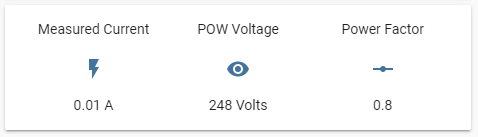Power factor input integra as a input Boolean, initial default 0.8,

AC single phase amps to watts calculation

The power P in watts (W) is equal to the PF times the phase current I in amps (A), times the RMS voltage V in volts (V):

P (W) = PF × I (A) × V (V)

Watts to kWh calculation

E (kWh) = P (W) × t (hr) / 1000

Would appreciate any assistance?

Regards,

1 Like

Yep, you have the right idea.

state_attr will get the attribute ‘X’ of the entity id. So your use of it to get the Amps would be correct assuming the amps were stored as the value ‘A’.

Don’t look at lovelace to see state names or attributes. Those are manipulated by many factors and are rarely the correct names of the states (state names aren’t as friendly usually). Instead, go to (Developer Tools > States) and under “Current Entities”, type in the name of the entity you want to check out. In this case “sensor.pow_voltage” probably.

There, you will see the state and any attributes it has. Those are the exact things you need to use in your templates.

Looking at your mqtt sensor, the state is the voltage. So you would use "states(‘entity_id’) to get the state value (as opposed to state_attr(‘entity_id’). Please notice the quotes used around the entity_id…this is important.

Lastly, with templates, everything inside of {{ }} will be evaluated, and everything oustide of those is simply a string.

So in your example, assuming we fixed the state_attr and states and other syntax, your template would evaluate from:

``````{{ states('input_number.power_factor') | int  }} x {{ states('sensor.pow_voltage') | int }} x {{ states('sensor.measured_current') | int  }}
``````

To (making up state numbers):

``````".11 x 110 x 10"
``````

i.e. the x (or * if you used the correct symbol for multiplication) would be printed as a string rather than evaluated).

``````# Example configuration.yaml entry
sensor:
- platform: template
sensors:
Kw:
friendly_name: "Kw"
unit_of_measurement: 'Kw'
value_template: {{ (states('input_number.power_factor') | int ) * (states('sensor.pow_voltage') | int) * (states('sensor.measured_current') | int)  }}
``````

KWh is going to be tougher as this will require you to keep track of your total usage for an entire hour. If you just want the instantaneous measurement, assuming constant load over the whole hour, it’s simple enough. Add this right below your Kw template sensor.

``````  - platform: template
sensors:
kWh:
friendly_name: "kWh"
unit_of_measurement: 'kWh'
value_template: {{ (states('sensor.kw') | int ) / 1000 }}
``````

This isn’t very exciting, it’s just your current usage converted into kW.

If you want something like a running average of your power usage, you can use a statistics sensor to keep track of it for you.

``````sensor:
- platform: statistics
name: kW_daily
entity_id: sensor.kw
sampling_size: 100
max_age:
hours: 24
``````

Now with this sensor, we can get a more meaningful kWh value as we can use the average kw over the entire last 24 hours.

``````  - platform: template
sensors:
kWh:
friendly_name: "kWh"
unit_of_measurement: 'kWh'
# kW_daily is a 24 hour average, so use 24 hours as the time value.
value_template: {{ (state_attr('sensor.kw_daily', 'mean') | int ) * 24 / 1000 }}
``````

You can tweak these numbers however you wish to get things that matter to you.

1 Like

Make a template sensor for the W. You already have the equation and it’s written here enough.

``````  - platform: template
sensors:
power_watts:
friendly_name: "Power Watts"
unit_of_measurement: 'W'
value_template: >
{% set PF = state_attr(input_number.power_factor.state | int ) %}
{% set V = state_attr('sensor.pow_voltage', 'V') %}
{% set A = state_attr('sensor.measured_current', 'A') %}
{{ PP * A * V }}
``````

Now, to properly calculate kWh, you want to use the integration integration.

This is where things get tricky. There’s a field called method. Choosing the correct method is all about math. I’ll make a quick synopsis of the styles:

## trapezoidal

This method averages 2 points of data and gets the area under the curve. See image below.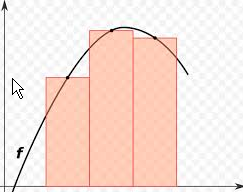Pros:

• Accurate if your data is constantly changing.

Cons:

• Not accurate if your data stays the same value for prolonged periods of time. If the next piece of data is wildly different, it will add a ton of error.

I recommend only using this method if your data is always changing and it has a ‘roll’ to it like a sine wave.

``````sensor:
- platform: integration
source: sensor.power_watts
name: Energy Spent
unit_prefix: k
method: trapezoidal
round: 2
``````

## left / right

This method chooses a point on the line and assumes it’s that value until the next point is received. For right, it’s reversed.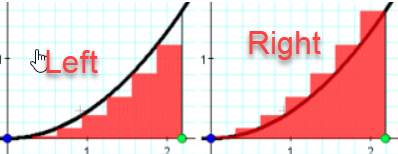Pros:

• Accurate if you have prolonged periods of data spikes (Think square waves).

Cons:

• When rising at a slow rate, the area under the curve always contains error. Positive error (right), or negative error(left). This is opposite when falling at a slow rate.
``````sensor:
- platform: integration
source: sensor.power_watts
name: Energy Spent
unit_prefix: k
method: left
round: 2

- platform: integration
source: sensor.power_watts
name: Energy Spent
unit_prefix: k
method: right
round: 2
``````

tldr:
Use left/right if you have momentary watt spikes like a square wave. Use trapezoidal when you have rounding peaks and valleys.

6 Likes

Hi,

I still have an issue, the sensor.power_watts returns back unavailable so I guess it is something to do with the way I am expressing my states?

``````  - platform: template

sensors:

power_watts:

friendly_name: "Power Watts"

unit_of_measurement: 'W'

value_template: >

{% set PF = state_attr(input_number.power_factor.state | int ) %}

{% set V = state_attr('sensor.pow_voltage', 'Volts') %}

{% set A = state_attr('sensor.measured_current', 'A') %}

{{ PP * A * V }}
``````

These are the screenshots from my developer tools / states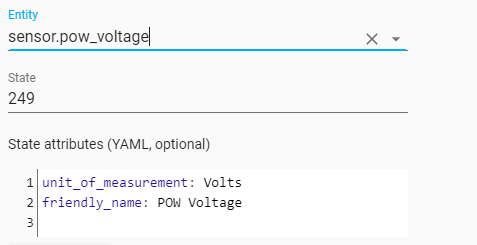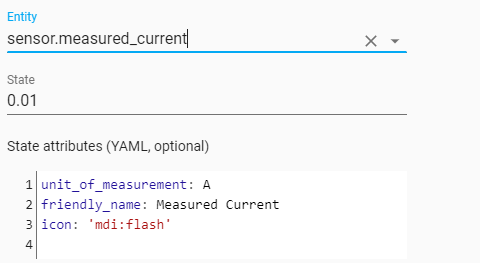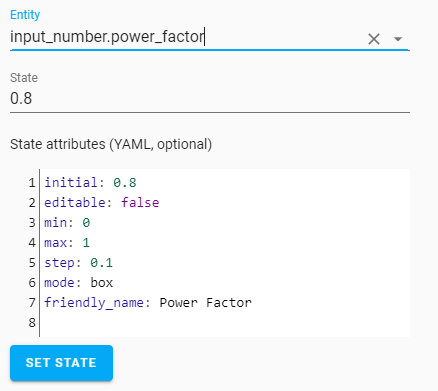And this is what I get.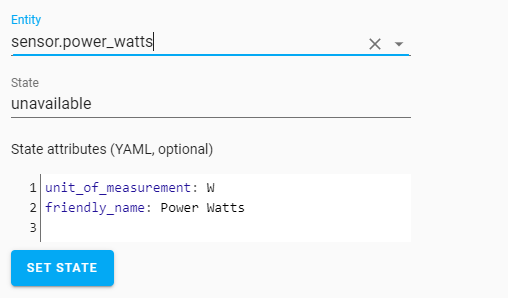From your example I noticed a small error I think, {{ PP * A * V }}, I changed it to PF but it still remains unavailable, for testing I set current to 5 for testing as my CT is not hooked up yet.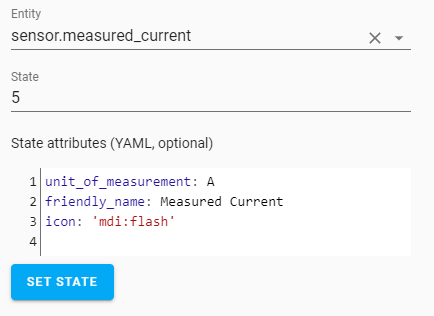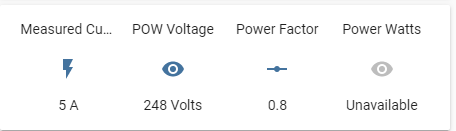Regards,

I donno what you were trying to access. state_attr is for getting attributes. You’re just trying to get the state.

``````  - platform: template

sensors:

power_watts:

friendly_name: "Power Watts"

unit_of_measurement: 'W'

value_template: >

{% set PF = states('input_number.power_factor') | int ) %}

{% set V = states('sensor.pow_voltage') | float %}

{% set A = states('sensor.measured_current') | float %}

{{ PF * A * V }}
``````

Petro,

Got it working

``````          {% set PF = states('input_number.power_factor') | float %}
``````

returns

missed comma between flow collection entries at line 538, column 65:
… e_attr(‘sensor.kw_daily’, ‘mean’) | int ) * 24 / 1000 }}
^

Any ideas?

This is YAML for now.

``````

- platform: template
sensors:
power_watts:
friendly_name: "Power Watts"
unit_of_measurement: 'W'
value_template: >

{% set PF = states('input_number.power_factor') | float %}

{% set V = states('sensor.pow_voltage') | float %}

{% set A = states('sensor.measured_current') | float %}

{{ PF * A * V }}

- platform: template
sensors:
kwh:
friendly_name: "KWh"
unit_of_measurement: 'KWh'
value_template: >

{% set W = states('input_number.power_watts') | float %}

{{ W / 1000 }}

- platform: integration
source: sensor.power_watts
name: Energy Spent
unit_prefix: k
method: trapezoidal
round: 2

- platform: statistics
name: kW_daily
entity_id: sensor.power_watts
sampling_size: 100
max_age:
hours: 24

- platform: template
sensors:
kwh:
friendly_name: "kWh"
unit_of_measurement: 'kWh'
# kW_daily is a 24 hour average, so use 24 hours as the time value.
value_template: {{ (state_attr('sensor.kw_daily', 'mean') | int ) * 24 / 1000 }}
``````

Again, thanks for your help and understanding.

Regards,

I would recommend using the integration integration (ha) that @petro suggested instead of the statistics one honestly.

As for the error, it would need quotes.

value_template: “{{ (state_attr(‘sensor.kw_daily’, ‘mean’) | int ) * 24 / 1000 }}”

Still no luck,

I copped and pasted and got

Invalid config for [sensor.template]: invalid template (TemplateSyntaxError: unexpected char ‘‘’ at 16) for dictionary value @ data[‘sensors’][‘kwh’][‘value_template’]. Got ‘“{{ (state_attr(‘sensor.kw_daily’, ‘mean’) | int ) * 24 / 1000 }}”’. (See ?, line ?).

you copied and pasted from the forum and the character set for the quotes is wrong. Read the error, it tells you the exact character it failed on. Character 16, which is the first quotation.

Also, just to clarify. The method you are attempting to use will be wrong at most times. You should really use the integration sensor. It was literally built to convert W to kWh. So the math is much better.

Implemented and all now working, many thanks

``````        value_template: "{{ (state_attr('sensor.kw_daily' , 'mean') | int ) * 24 / 1000 }}"
``````

I don’t know if you’re purposely ignoring or what. You should be using the integration sensor. Then once you have that sensor, use the utility integration. It will do all the math correctly instead of your flat out wrong calculation. People built these tools so that you don’t have to do the math and you get the correct result. Your hourly results will be so far off from reality and they will be completely wrong for the first 24 hours, while being mostly wrong the remaining time. Not to mention, you won’t be able to use utility meter because you have these wrong results! I feel like i’m taking crazy pills! But if you’re okay with bad results, to each their own I guess…

3 Likes

I wasn’t aware of ignoring you, I have implemented the code you have provided me with and also configured the utility integration as follows.

## Configuration

To enable the Utility Meter Sensor in your installation, add the following to your `configuration.yaml` file:

``````# Example configuration.yaml entry
utility_meter:
energy:
source: sensor.energy_in_kwh
cycle: monthly
``````

in my case I have the following.

``````  - platform: template
sensors:
power_watts:
friendly_name: "Power Watts"
unit_of_measurement: 'W'
value_template: >

{% set PF = states('input_number.power_factor') | float %}

{% set V = states('sensor.pow_voltage') | float %}

{% set A = states('sensor.measured_current') | float %}

{{ PF * A * V }}

- platform: template
sensors:
power_kw:
friendly_name: "Power KW"
unit_of_measurement: 'KW'
value_template: >

{% set W = states('input_number.power_watts') | float %}

{{ W / 1000 }}

- platform: integration
source: sensor.power_watts
name: Energy Spent
unit_prefix: k
method: trapezoidal
round: 2

- platform: statistics
name: kW_daily
entity_id: sensor.power_watts
sampling_size: 100
max_age:
hours: 24

utility_meter:
energy:
source: sensor.energy_spent
cycle: monthly
``````

So my understanding is I take the sensorr.energy_spent for the utility meter source?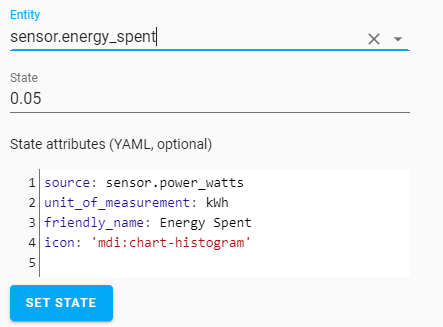Again, sorry if I went off course for a while I just wanted to see how to implement the code, after all I am learning by doing…

Regards,

Yeah, he’s saying do not use the statistics sensor I suggested because It is the wrong thing to use for this scenario.

Just remove the statistics sensor completely (sensor.kW_daily). The integration one will actually provide a meaningful number.

It just felt like you were ignoring because you kept asking questions on how to fix the statistics.kw_daily syntax. It probably wasn’t clear that his solution was a straight up replacement to that, so you asking those questions made it seem like you weren’t using the integration one!

Hi, In trying to round the output to two decimal places, I have tried the following but it doesn’t work.

``````  - platform: template
sensors:
power_watts:
friendly_name: "Power Watts"
unit_of_measurement: 'W'
value_template: >

{% set PF = states('input_number.power_factor') | float %}

{% set V = states('sensor.pow_voltage') | float %}

{% set A = states('sensor.measured_current') | float %}

{{ PF * A * V |round(2) }}
``````

Any ideas?

Regards,

``````{{ (PF * A * V) |round(2) }}
``````

Thanks, worked a treat

Hey petro, sorry for necroposting but just had a thought about minimising the error due to choosing left or right integration method.

Would there be any pitfalls in creating two integral sensors, one left and one right and taking the average of the two?

Pretty sure you’d get the same answer as the trapezoidal. The ‘real’ problem is that it would need to act on information when at zero. I have no idea why that wasn’t thought of. But the fact that you can get the same state change 9000 times and it only appear as 1 data points drastically skews the math for integration.

1 Like

Is there a way to have the integration sensor work off historical values so that when I restart home assistant it doesn’t lose the total?

The integration sensor only works off historical data.